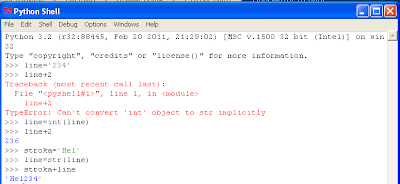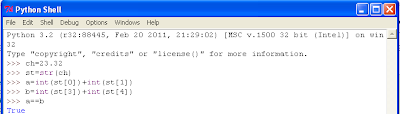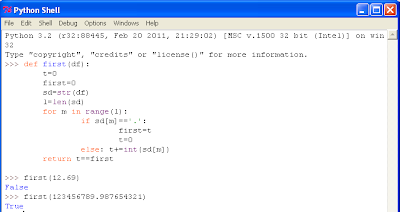# String conversion in Python

In order to convert a number to a string in Python, we use the str() function, and to convert a string to a number, we use int() function (pic. 1)Picture 1. Data conversion

Firstly, we create a string ‘234’ (pic. 1) and then add 2 to it. But the addition does not happen because of the data types’ difference. When we convert ‘234’ into number 234, now we can add 2. Concatenation works by the following principle.

Bearing in mind these two functions, we can solve the following task. A user inputs a number, i.e. 78,23. In case the sum of numbers before the comma and after are equal, then the application outputs TRUE, if not – FALSE.Picture 2. Solving the task in a primitive way

To solve the task, we will create a variable with a numeric value (for example, 23.32), then convert the data from the digital to the string type and assign the new value to the variable st. Then knowing that before comma two characters can be converted into numbers and added using the int() function. We will make the same with the numbers after the comma. The last action is to check whether those two variables containing the sum of the number before and after the comma are equal.

Picture 3 shows the working application code (Function first) which requires only the digital value and then it outputs the result.Picture 3. Function first()

This function converts numbers into a string, then calculates the number of symbols in a string with the help of len() function. Then, through for and range() function, the application compares each symbol and if it is not ‘.’, then it converts the symbol into a number and assigns the t variable value. It happens until the application does not meet a ‘.’. After ‘.’, the sum of the numbers before ‘.’ is assigned to the new variable first = t, and the sum of the numbers after ‘.’ is assigned to the same variable t but from the beginning assigning to it the value 0 to reset the variable. As soon as the cycle reaches the end of the word sd, the obtained variables are compared and the result of the comparison is output on the screens.

Tags: